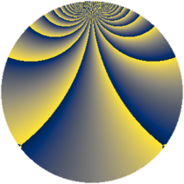# Properties

 Label 1815.2.xLevel $1815$ Weight $2$ Character orbit 1815.x Rep. character $\chi_{1815}(112,\cdot)$ Character field $\Q(\zeta_{20})$ Dimension $864$ Sturm bound $528$

# Related objects

## Defining parameters

 Level: $$N$$ $$=$$ $$1815 = 3 \cdot 5 \cdot 11^{2}$$ Weight: $$k$$ $$=$$ $$2$$ Character orbit: $$[\chi]$$ $$=$$ 1815.x (of order $$20$$ and degree $$8$$) Character conductor: $$\operatorname{cond}(\chi)$$ $$=$$ $$55$$ Character field: $$\Q(\zeta_{20})$$ Sturm bound: $$528$$

## Dimensions

The following table gives the dimensions of various subspaces of $$M_{2}(1815, [\chi])$$.

Total New Old
Modular forms 2304 864 1440
Cusp forms 1920 864 1056
Eisenstein series 384 0 384

## Trace form

 $$864q + 8q^{5} + 20q^{7} + O(q^{10})$$ $$864q + 8q^{5} + 20q^{7} + 16q^{12} + 12q^{15} + 248q^{16} + 20q^{17} + 60q^{20} - 32q^{23} + 32q^{25} + 16q^{26} + 60q^{28} + 40q^{30} - 16q^{31} + 216q^{36} - 8q^{37} - 56q^{38} + 120q^{41} - 12q^{42} + 200q^{46} - 60q^{47} - 48q^{48} - 80q^{50} - 40q^{51} - 40q^{52} - 36q^{53} + 16q^{56} - 40q^{57} - 20q^{58} - 48q^{60} - 40q^{61} - 80q^{62} - 20q^{63} + 112q^{67} - 80q^{68} + 108q^{70} - 64q^{71} + 60q^{73} + 16q^{75} + 192q^{78} + 40q^{80} + 216q^{81} - 56q^{82} + 200q^{83} + 80q^{85} + 96q^{86} - 88q^{91} - 60q^{92} + 72q^{93} - 60q^{95} - 32q^{97} + O(q^{100})$$

## Decomposition of $$S_{2}^{\mathrm{new}}(1815, [\chi])$$ into newform subspaces

The newforms in this space have not yet been added to the LMFDB.

## Decomposition of $$S_{2}^{\mathrm{old}}(1815, [\chi])$$ into lower level spaces

$$S_{2}^{\mathrm{old}}(1815, [\chi]) \cong$$ $$S_{2}^{\mathrm{new}}(55, [\chi])$$$$^{\oplus 4}$$$$\oplus$$$$S_{2}^{\mathrm{new}}(165, [\chi])$$$$^{\oplus 2}$$$$\oplus$$$$S_{2}^{\mathrm{new}}(605, [\chi])$$$$^{\oplus 2}$$